# How to Calculate and Solve for Shear Stress | Material Selection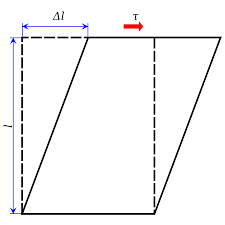The image above represents shear stress.

To compute for shear stress, three essential parameters are needed and these parameters are Twisting Moment (M), Radius (r) and Polar Moment of Inertia (J).

The formula for calculating shear stress:

τ = Mr/J

Where:

τ = Shear Stress
M = Twisting Moment
J = Polar Moment of Inertia

Let’s solve an example;
Find the shear stress when the twisting moment is 12, the radius is 8 and the polar moment of inertia is 14.

This implies that;

M = Twisting Moment = 12
J = Polar Moment of Inertia = 14

τ = Mr/J
τ = (12)(8)/14
τ = 96/14
τ = 6.85

Therefore, the shear stress is 6.85 Pa.

Calculating the Twisting Moment when the Shear Stress, the Radius and the Polar Moment of Inertia is Given.

M = τJ / r

Where;

M = Twisting Moment
τ = Shear Stress
J = Polar Moment of Inertia

Let’s solve an example;
Find the twisting moment when the shear stress is 10, the radius is 6 and the polar moment of inertia is 4.

This implies that;

τ = Shear Stress = 10
J = Polar Moment of Inertia = 4

M = τJ / r
M = 10 x 4 / 6
M = 40 / 6
M = 6.67

Therefore, the twisting moment is 6.67.

Calculating the Radius when the Shear Stress, the Twisting Moment and the Polar Moment of Inertia is Given.

r = τJ / M

Where;

τ = Shear Stress
M = Twisting Moment
J = Polar Moment of Inertia

Let’s solve an example;
Find the radius when the shear stress is 8, the twisting moment is 4 and the polar moment of inertia is 3.

This implies that;

τ = Shear Stress = 8
M = Twisting Moment = 4
J = Polar Moment of Inertia = 3

r = τJ / M
r = 8 x 3 / 4
r = 24 / 4
r = 6

Calculating the Polar Moment of Inertia when the Shear Stress, the Twisting Moment and the Radius is Given.

J = Mr / τ

Where;

J = Polar Moment of Inertia
τ = Shear Stress
M = Twisting Moment

Let’s solve an example;
Find the polar moment of inertia when the shear stress is 12, the twisting moment is 8 and the radius is 4.

This implies that;

τ = Shear Stress = 12
M = Twisting Moment = 8

J = Mr / τ
J = 8 x 4 / 12
J = 32 / 12
J = 2.67

Therefore, the polar moment of inertia is 2.67.

Nickzom Calculator – The Calculator Encyclopedia is capable of calculating the shear stress.

To get the answer and workings of the shear stress using the Nickzom Calculator – The Calculator Encyclopedia. First, you need to obtain the app.

You can get this app via any of these means:

To get access to the professional version via web, you need to register and subscribe for NGN 2,000 per annum to have utter access to all functionalities.
You can also try the demo version via https://www.nickzom.org/calculator

Apple (Paid) – https://itunes.apple.com/us/app/nickzom-calculator/id1331162702?mt=8
Once, you have obtained the calculator encyclopedia app, proceed to the Calculator Map, then click on Materials and Metallurgical under Engineering.Now, Click on Material Selection under Materials and Metallurgical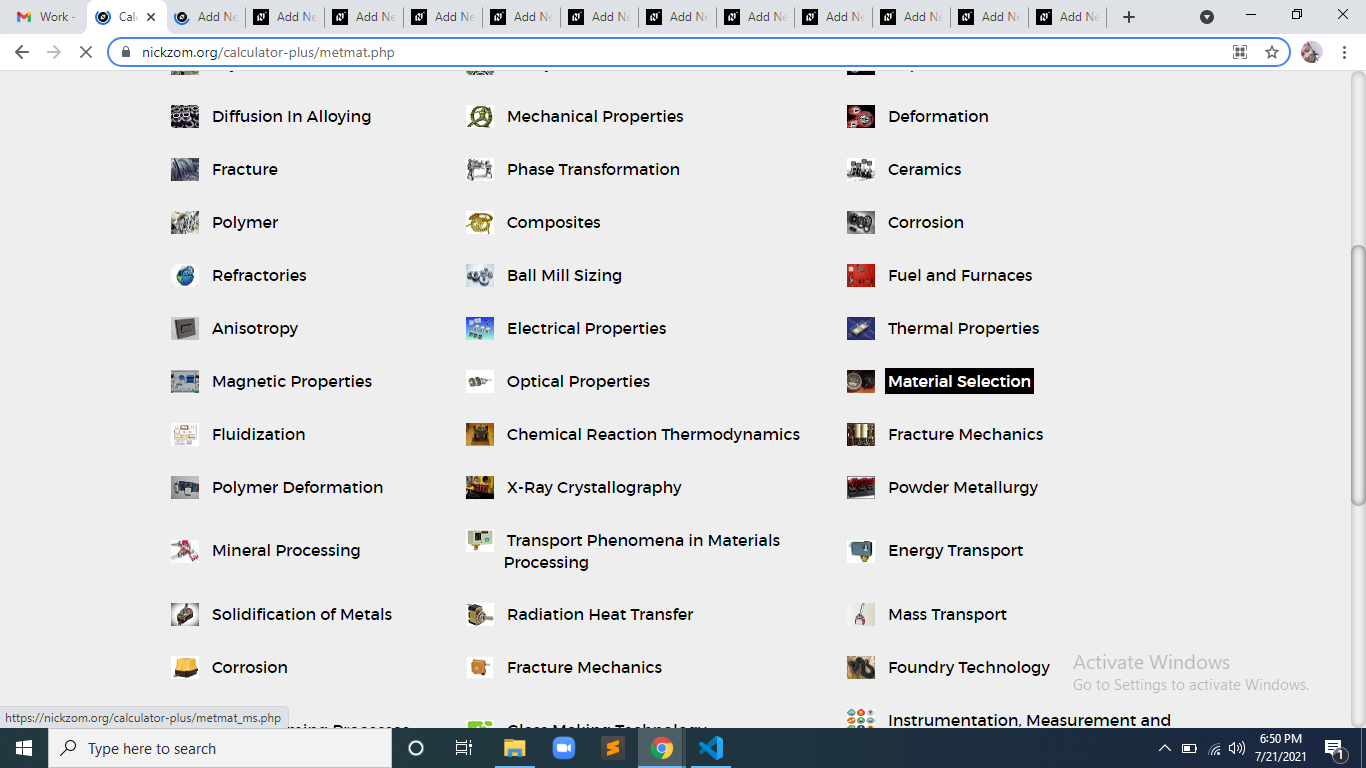Now, Click on Shear Stress under Material Selection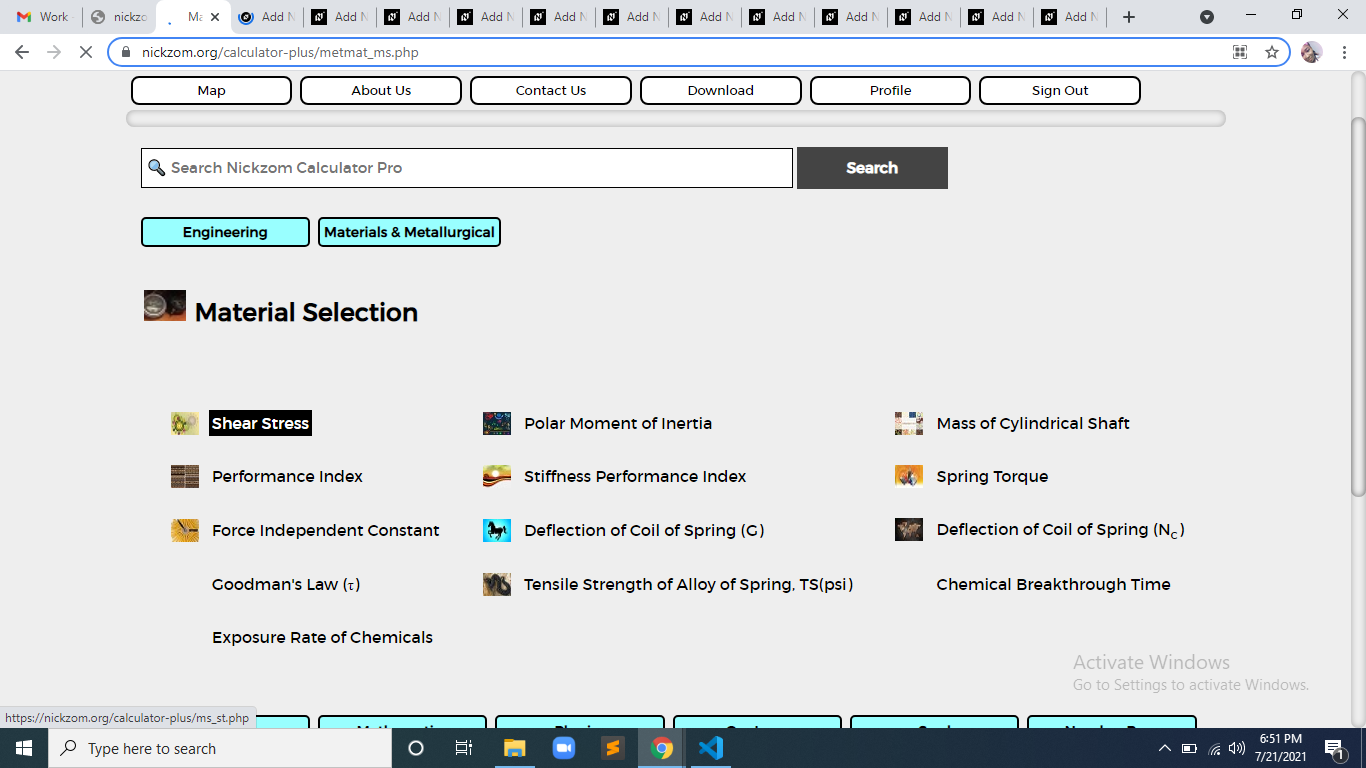The screenshot below displays the page or activity to enter your values, to get the answer for the shear stress according to the respective parameters which is the Twisting Moment (M), Radius (r) and Polar Moment of Inertia (J).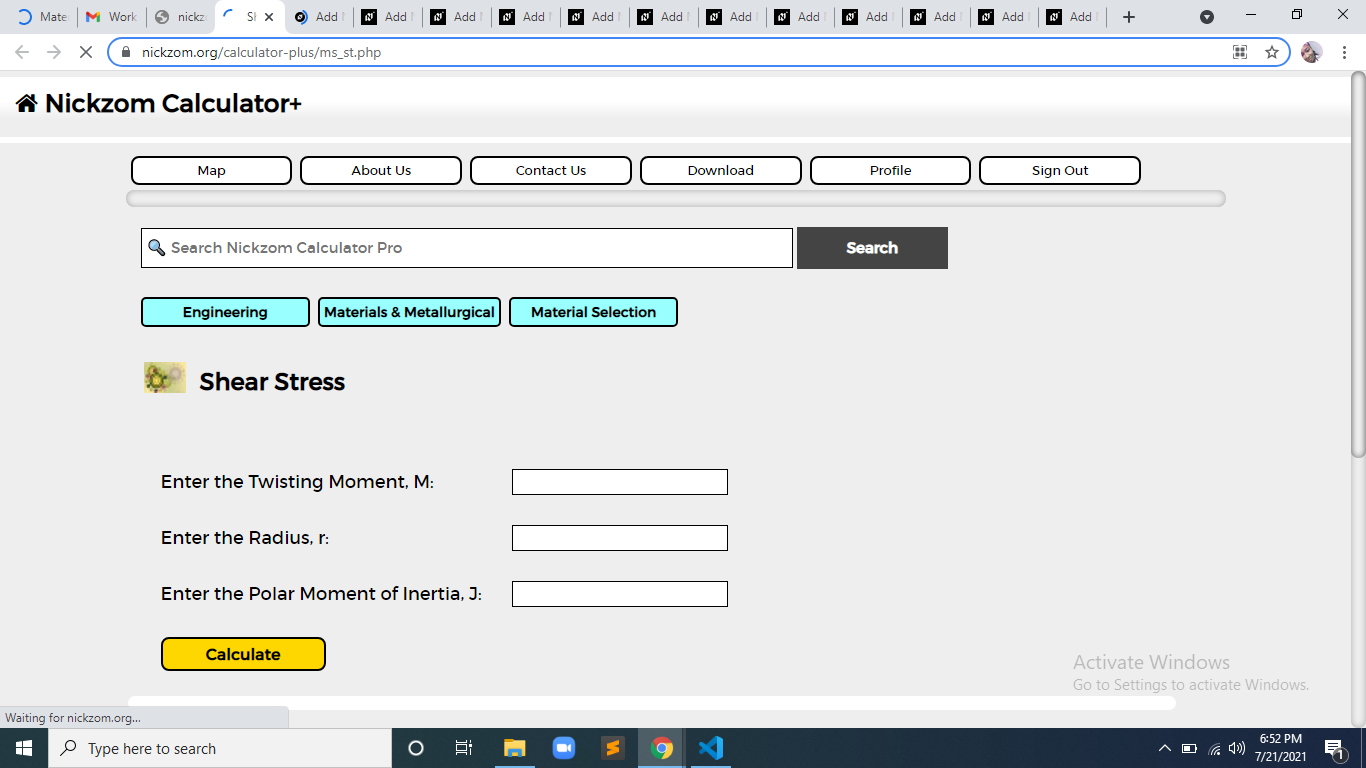Now, enter the values appropriately and accordingly for the parameters as required by the Twisting Moment (M) is 12, Radius (r) is 8 and Polar Moment of Inertia (J) is 14.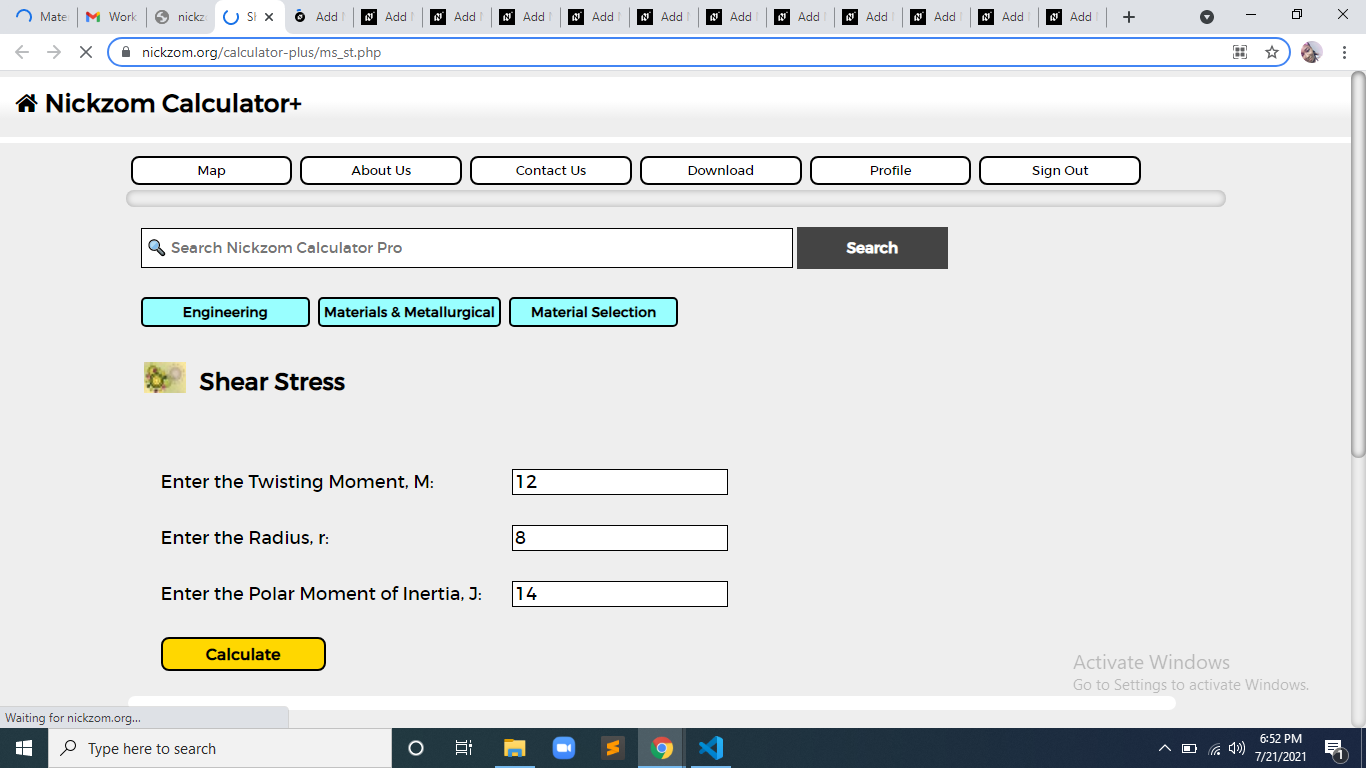Finally, Click on Calculate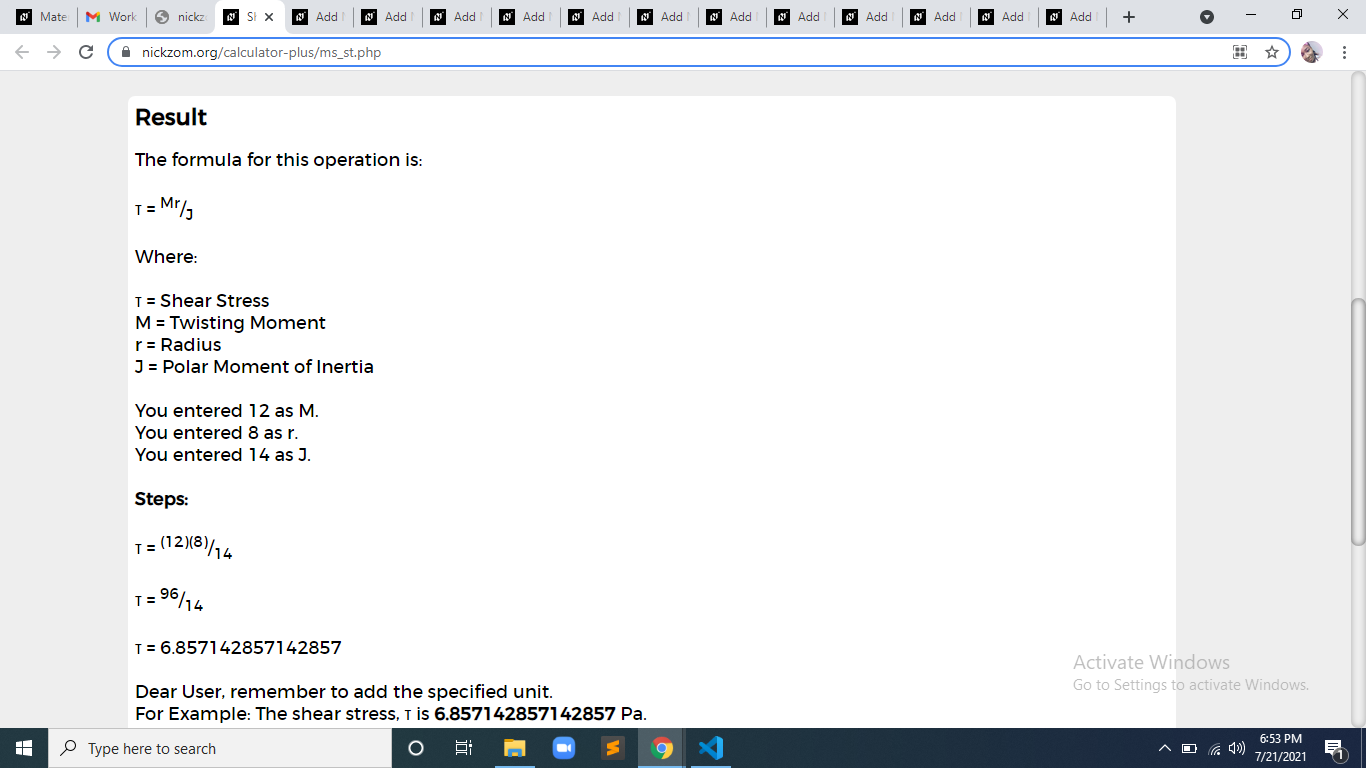As you can see from the screenshot above, Nickzom Calculator– The Calculator Encyclopedia solves for the shear stress and presents the formula, workings and steps too.